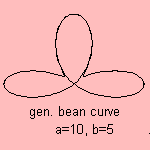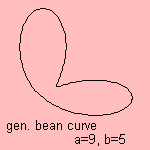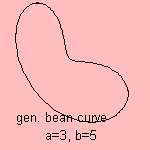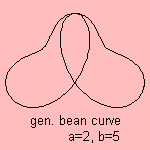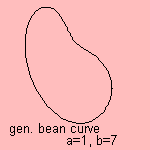# generalized bean curve

## higher

last updated: 2004-05-05The curve is a generalization of the bean curve (a=b=3).
For a=b=1 and a=b=2 the curve is the circle.

We see the following:

• the bean curvea, b is equivalent to the bean curveb, a
• the order of the curve is max(a,b) (a and b both uneven) or 1/2 max (a,b) (a or b even)
• when a or b is even, the curve is compound of two symmetric bean curves
• the values for a and b define how much the curve is pressed in from a circle towards a right angle figure, a for the vertical part, b for the horizontal part